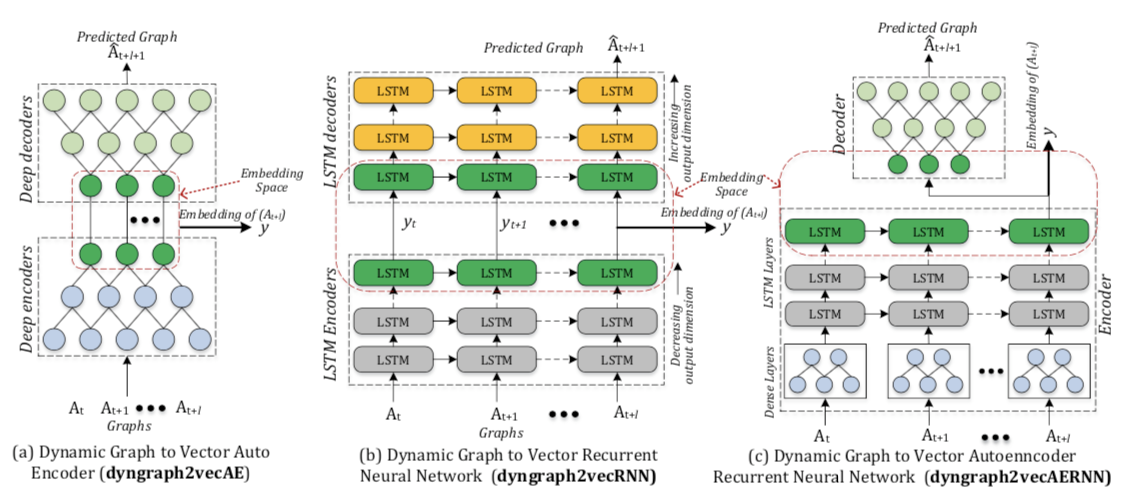# dyngraph2vec:Capturing Network Dynamics using Dynamic Graph Representation Learning

## 方法

### 问题陈述

• 图：$G(V, E)$
• 动态图：$\mathcal{G}={G_1,…,G_T}$
• 目标：将一个节点，通过学习一个映射方法：$f_t=\lbrace V_1,…,V_t,E_1,…,E_t\rbrace \to \Bbb{R}^d$转换为一组低维向量：$y_{v_1},…,y_{v_t}$，其中$y_{v_i}=f_i(V_1,…,V_i,E_1,…,Ei)$，是一个 d 维的向量，捕获了$1-i$时刻的图动态变换。

#### dyngraph2vec

dyngraph2vec 是一个深度学习模型，输入前面几个时间片段的图，即可输出下一个时刻的图。

$\mathcal{B}$是惩罚项，$\mathcal{B}_{ij}=\beta, for (i,j) \in E_{t+l+1}, else 1$。

$\odot​$表示一种矩阵的布尔运算，具体定义可以在这个pdf里面找一下（https://www.math.fsu.edu/~pkirby/mad2104/SlideShow/s5_4.pdf），不再详述。